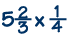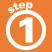Home    |    Teacher    |    Parents    |    Glossary    |    About UsHere are the steps for multiplying mixed numbers.

1. Change each number to an improper fraction.
2. Simplify if possible.
3. Multiply the numerators and then the denominators.
4. Put answer in lowest terms.
5. Check to be sure the answer makes sense.

Click on each step below to see an example.Homework Help | Pre-Algebra | FractionsEmail this page to a friendSearch·  Definitions·  Reducing fractions·  Adding and subtract-     ing fractions·  Multiplying fractions·  Dividing fractions·  Adding and subtract-     ing mixed numbers·  Multiplying     mixed numbers·  Dividing     mixed numbersFirst Glance In Depth Examples WorkoutMultiplying mixed numbers